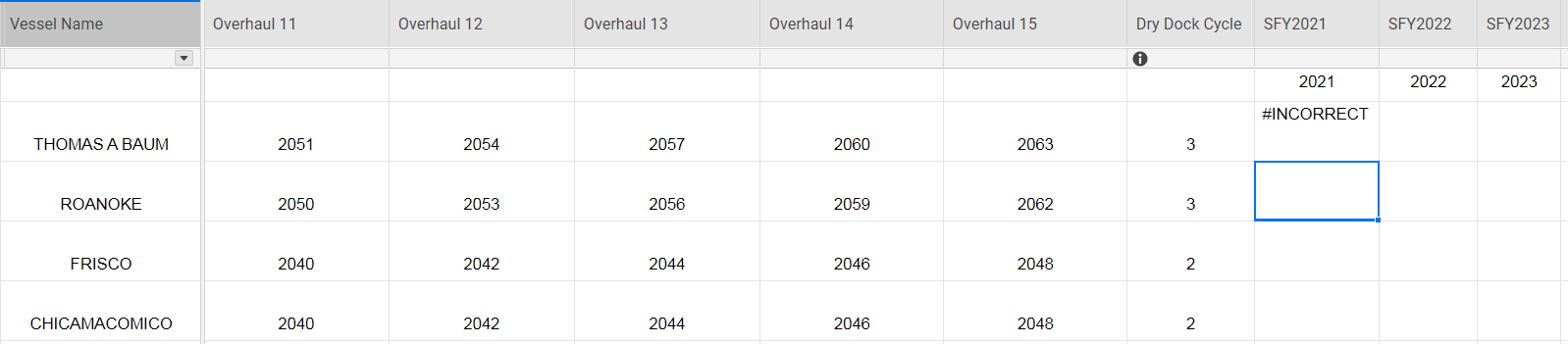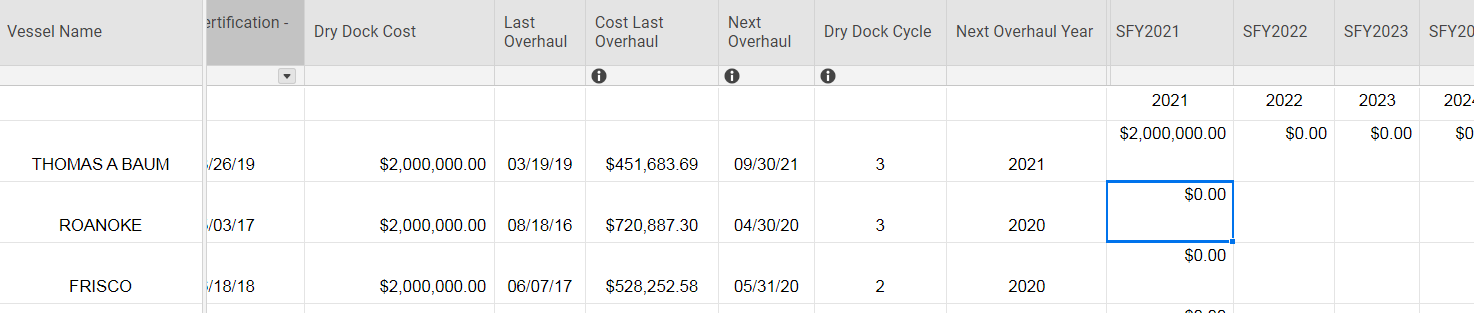# SUMIF Formula

Working on a project where I want to put a total in a column based on if an asset needs a rehab.  I would like for the formula to find the year and if it matches with the column, put the total in that cell.

Here's what I've got:

=SUMIF([Next Overhaul Year]2:[Overhaul 15]2, =[SFY2021]1, [Dry Dock Cost]@row)

Getting an invalid argument set error message.

Here's a screen shot for reference:• https://help.smartsheet.com/function/sumif

---------------------------------------------------------------------------------

=SUMIF(range, criterion,[sum_range])

Range: The range to analyze

Criterion: The criteria for said range

[sum_range]: in hard brackets because it is optional. If included, this will be the range summed up. otherwise, first range will be summed.

*(The number of cells in both ranges must be equal to each other)

----------------------------------------------------------------------------------

Beyond that, I cannot write your formula as I don't understand how you are associating the date with the values.

• I'm trying to put a value in a cell if any of those years in that range equals the year in the column. Does that help?

• So the sum range is where I'm going wrong.  The "range" for me is just a single cell.  So, I regrouped and tried this formula:

=IFERROR(IF(MATCH(2021, [Next Overhaul Year]@row:[Overhaul 15]@row), [Dry Dock Cost]@row, 0), 0)

It works for the first cell, but when I drag it down, it's not working properly.

• Try using a CONTAINS function within an IF statement.

=IF(CONTAINS(2021, [Next Overhaul Year]@row:[Overhaul 15]@row), [Dry Dock Cost]@row)

• That didn't work either.  Lemme try a different approach and post below.

• I'm trying to budget out how much it will cost to pay for dry docks for each boat each year.  The cost is set and I have the date for the next scheduled dry dock and each boat is on a 2, 3, or 5 year cycle, depending on the boat. Is there a way to project out by year based on the dry dock cycle? I started with this formula:

=SUMIF([Next Overhaul Year]2, [SFY2021]\$1, [Dry Dock Cost]@row)

So, I want to calculate for 2022 - 2050.  Screenshot below for reference.• =SUMIF([Next Overhaul Year]:[Next Overhaul Year], 2021, [Dry Dock Cost]:[Dry Dock Cost])

This will work for 2021, but it will be a pain to drag it, as you will have to type it in every time. Also you will have a long list of historical projections I would imagine are rather useless. I would name the columns you currently have posted as the year as 1-30 or something similar so it isn't confusing when the years don't match up, then use this formula in the first row:

=year(today())+1

Then post the expected results in the second row as the formula

=SUMIF([Next Overhaul Year]:[Next Overhaul Year], year(today())+count(\$1:1), [Dry Dock Cost]:[Dry Dock Cost])

or something similar. This way you can drag the formula easily, and it will always show the next 30 years.